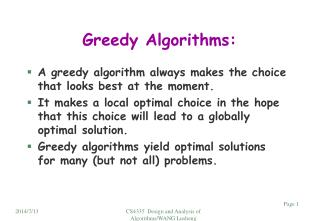# Greedy Algorithms: - PowerPoint PPT PresentationDownload PresentationGreedy Algorithms:

Greedy Algorithms:Download Presentation## Greedy Algorithms:

- - - - - - - - - - - - - - - - - - - - - - - - - - - E N D - - - - - - - - - - - - - - - - - - - - - - - - - - -
##### Presentation Transcript

1. Greedy Algorithms: • A greedy algorithm always makes the choice that looks best at the moment. • It makes a local optimal choice in the hope that this choice will lead to a globally optimal solution. • Greedy algorithms yield optimal solutions for many (but not all) problems. CS4335 Design and Analysis of Algorithms/WANG Lusheng

2. The 0-1 Knapsack problem: • The 0-1 knapsack problem: • N items, where the i-th item is worth vi dollars and weight wi pounds. 11 p 3 p 4p 58 p 8p 88p • vi and wi are integers. 3\$ 6 \$ 35\$ 8\$ 28\$ 66\$ • We can carry at most W (integer) pounds. • How to take as valuable a load as possible. • An item cannot be divided into pieces. • The fractional knapsack problem: • The same setting, but the thief can take fractions of items. • W may not be integer. W CS4335 Design and Analysis of Algorithms/WANG Lusheng

3. Solve the fractional Knapsack problem: • Greedy on the value per pound vi/wi. • Each time, take the item with maximum vi/wi . • If exceeds W, take fractions of the item. • Example:(1, 5\$), (2, 9\$), (3, 12\$), (3, 11\$) and w=4. • vi/wi : 5 4.5 4.0 3.667 • First: (1, 5\$), Second: (2, 9\$), Third: 1/3 (3, 12\$) • Total W: 1, 3, 4. Can only take part of item CS4335 Design and Analysis of Algorithms/WANG Lusheng

4. Proof of correctness: (The hard part) X i1 w1 i2 w2wj . . . ip wp . . . ikwk Let X = i1, i2, …ik be the optimal items taken. • Consider the item j : (vj, wj)with the highest v/w. • if j is not used in X (the optimal solution), get rid of some items with total weight wj (possibly fractional items) and add item j. (since fractional items are allowed, we can do it.) • Total value is increased. Why? • One more item selected by greedy is added to X • Repeat the process, X is changed to contain all items selected by greedy WITHOUT decreasing the total value taken by the thief. CS4335 Design and Analysis of Algorithms/WANG Lusheng

5. The 0-1 knapsack problem cannot be solved optimally by greedy • Counter example: (moderate part) • W=10 21.8 • Items found (6pounds, 12dollars), (5pounds, 9 dollar), 1.8 1. 1 (5pounds, 9 dollars), (3pounds, 3 dollars), (3 pounds, 3 dollars) • If we first take (6, 12) according to greedy algorithm, then solution is (6, 12), (3, 3) (total value is 12+3=15). • However, a better solution is (5, 9), (5, 9) with total value 18. To showthata statement does not hold, we only have to give an example. CS4335 Design and Analysis of Algorithms/WANG Lusheng

6. A subset of mutually compatibles jobs: {c, f} CS4335 Design and Analysis of Algorithms/WANG Lusheng

7. CS4335 Design and Analysis of Algorithms/WANG Lusheng

8. CS4335 Design and Analysis of Algorithms/WANG Lusheng

9. Sorting the n jobs based on fi needs O(nlog n) time CS4335 Design and Analysis of Algorithms/WANG Lusheng

10. Example: • Jobs (s, f): (0, 10), (3, 4), (2, 8), (1, 5), (4, 5), (4, 8), (5, 6) (7,9). Sorting based on fi: (3, 4) (1, 5), (4, 5) (5, 6) (4,8) (2,8) (7, 9)(0,10). Selecting jobs: (3,4) (4, 5) (5,6) (7, 9) CS4335 Design and Analysis of Algorithms/WANG Lusheng

11. CS4335 Design and Analysis of Algorithms/WANG Lusheng

12. CS4335 Design and Analysis of Algorithms/WANG Lusheng

13. Sort ob finish time: b, c, a, e, d, f, g, h. Greedy algorithm Selects: b, e, h. CS4335 Design and Analysis of Algorithms/WANG Lusheng

14. Depth: The maximum No. of jobs required at any time. Depth:3 CS4335 Design and Analysis of Algorithms/WANG Lusheng

15. CS4335 Design and Analysis of Algorithms/WANG Lusheng

16. Depth: The maximum No. of jobs required at any time. CS4335 Design and Analysis of Algorithms/WANG Lusheng

17. Greedy on start time CS4335 Design and Analysis of Algorithms/WANG Lusheng

18. Greedy Algorithm: c d g j b f i Depth: The maximum No. of jobs required at any time. a e h Depth:3 CS4335 Design and Analysis of Algorithms/WANG Lusheng

19. ddepth CS4335 Design and Analysis of Algorithms/WANG Lusheng

20. CS4335 Design and Analysis of Algorithms/WANG Lusheng

21. CS4335 Design and Analysis of Algorithms/WANG Lusheng

22. l1=0, l2=1 l2=0, l1=0 0 11 10 l1=9, l2=0 l1=0, l2=1 1 0 11 CS4335 Design and Analysis of Algorithms/WANG Lusheng

23. CS4335 Design and Analysis of Algorithms/WANG Lusheng

24. CS4335 Design and Analysis of Algorithms/WANG Lusheng

25. di<dj We check every pair of consecutive numbers, if there is not inversion, then the whole sequenc has no inversion. n1n2 … nk Example: 1, 2, 3, 4, 5, 6, 7, 8, 9 1, 2, 6, 7, 3, 4, 5, 8, 9 CS4335 Design and Analysis of Algorithms/WANG Lusheng

26. di<dj CS4335 Design and Analysis of Algorithms/WANG Lusheng

27. CS4335 Design and Analysis of Algorithms/WANG Lusheng

28. Example: Job ti di • 2 2 • 3 4 • 4 6 • 4 8 • 6 10 j1 j2 5 9 13 j5 19 2 j3 j4 CS4335 Design and Analysis of Algorithms/WANG Lusheng

29. CS4335 Design and Analysis of Algorithms/WANG Lusheng